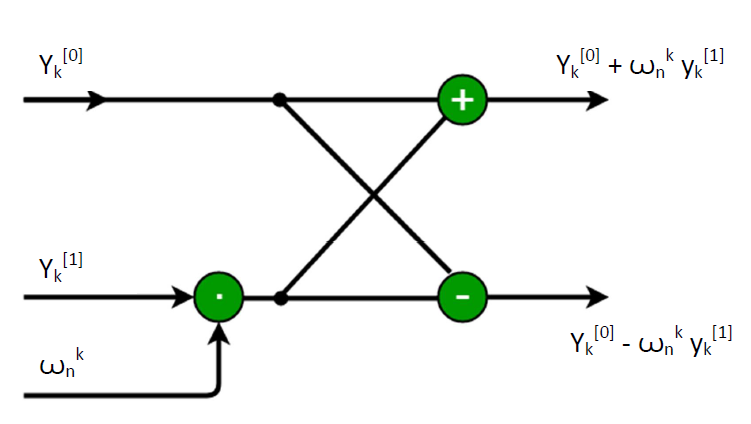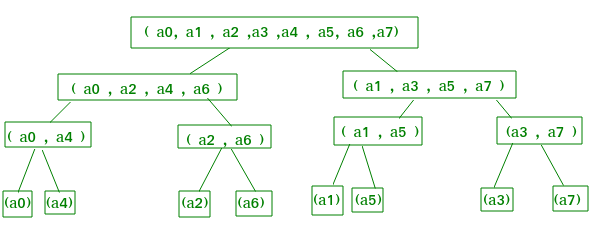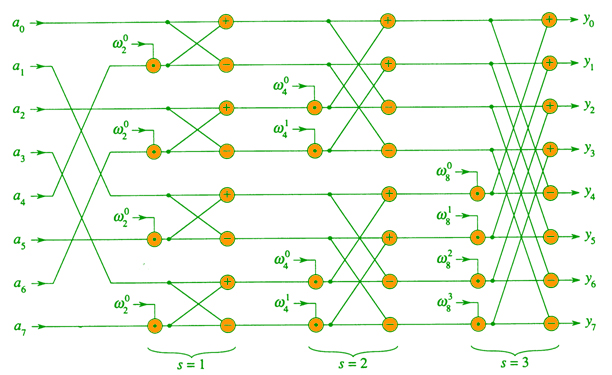# Iterative Fast Fourier Transformation for polynomial multiplication

Given two polynomials, A(x) and B(x), find the product C(x) = A(x)*B(x). In the previous post we discussed the recursive approach to solve this problem which has O(nlogn) complexity.
Examples:

Input :
A[] = {9, -10, 7, 6}
B[] = {-5, 4, 0, -2}
Output :
C(x) =## Recommended: Please try your approach on {IDE} first, before moving on to the solution.

In real life applications such as signal processing, speed matters a lot, this article examines an efficient FFT implementation. This article focuses on iterative version of the FFT algorithm that runs in O(nlogn) time but can have a lower constant hidden than the recursive version plus it saves the recursion stack space.
Pre-requisite: recursive algorithm of FFT.
Recall the recursive-FFT pseudo code from previous post, in the for loop evaluation of,is calculated twice. We can change the loop to compute it only once, storing it in a temporary variable t. So, it becomes,
for k0 to n/2 – 1
do tThe operation in this loop, multiplying the twiddle factor w =by, storing the product into t, and adding and subtracting t from, is known as a butterfly operation.
Pictorially, this is what butterfly operation looks like:Let us take for n=8 and procede for formation of iterative fft algorithm. Looking at the recursion tree above, we find that if we arrange the elements of the initial coefficient vector a into the order in which they appear in the leaves, we could trace the execution of the Recusive-FFT procedure, but bottom up instead of top down. First, we take the elements in pairs, compute the DFT of each pair using one butterfly operation, and replace the pair with its DFT. The vector then holds n/2 2-element DFTs. Next, we take these n/2 DFTs in pairs and compute the DFT of the four vector elements they come from by executing two butterfy operations, replacing two 2-element DFTs with one 4-element DFT. The vector then holds n/4 4-element DFTs. We continue in this manner until the vector holds two (n/2) element DFTs, which we combine using n/2 butterfly operations into the final n-element DFT.To turn this bottom-up approach into code, we use an array A[0…n] that initially holds the elements of the input vector a in the order in which they appear in the leaves of the tree. Because we have to combine DFT so n each level of the tree, we introduce avariable s to count the levels, ranging from 1 (at the bottom, when we are combining pairs to form 2-element DFTs) to lgn (at the top, when weare combining two n/2 element DFTs to produce the final result). The algorithm therefore is:

1. for s=1 to lgn
2.     do for k=0 to n-1 by3.           do combine the two-element DFTs in
A[k...k+-1] and A[k+...k+-1]
into one 2s-element DFT in A[k...k+-1]


Now for generating the code, we arrange the coefficient vector elements in the order of leaves. Example- The order in leaves 0, 4, 2, 6, 1, 5, 3, 7 is a bit reversal of the indices. Start with 000, 001, 010, 011, 100, 101, 110, 111 and reverse the bits of each binary number to obtain 000, 100, 010, 110, 001, 101, 011, 111.Pseudo code for iterative FFT :

BIT-REVERSE-COPY(a, A)
n = length [a]
for k = 0 to n-1
do A[rev(k)] = a[k]

ITERATIVE-FFT
BIT-REVERSE-COPY(a, A)
n = length(a)
for s = 1 to log n
do m==for j = 0 to m/2-1
do for k = j to n-1 by m
do t =A[k+m/2]
u = A[k]
A[k] = u+t
A[k+m/2] = u-treturn A


It will be more clear from the below parallel FFT circuit :// CPP program to implement iterative  // fast Fourier transform.  #include  using namespace std;     typedef complex<double> cd;  const double PI = 3.1415926536;     // Utility function for reversing the bits  // of given index x  unsigned int bitReverse(unsigned int x, int log2n)  {      int n = 0;      for (int i = 0; i < log2n; i++)      {          n <<= 1;          n |= (x & 1);          x >>= 1;      }      return n;  }     // Iterative FFT function to compute the DFT  // of given coefficient vector  void fft(vector& a, vector& A, int log2n)  {      int n = 4;         // bit reversal of the given array      for (unsigned int i = 0; i < n; ++i) {          int rev = bitReverse(i, log2n);          A[i] = a[rev];      }         // j is iota      const complex<double> J(0, 1);      for (int s = 1; s <= log2n; ++s) {          int m = 1 << s; // 2 power s          int m2 = m >> 1; // m2 = m/2 -1          cd w(1, 0);             // principle root of nth complex           // root of unity.          cd wm = exp(J * (PI / m2));           for (int j = 0; j < m2; ++j) {              for (int k = j; k < n; k += m) {                     // t = twiddle factor                  cd t = w * A[k + m2];                   cd u = A[k];                     // similar calculating y[k]                  A[k] = u + t;                      // similar calculating y[k+n/2]                  A[k + m2] = u - t;               }              w *= wm;          }      }  }     int main()  {      vector a{ 1, 2, 3, 4 };      vector A(4);      fft(a, A, 2);      for (int i = 0; i < 4; ++i)          cout << A[i] << "\n";  }

Input:  1 2 3 4
Output:
(10, 0)
(-2, -2)
(-2, 0)
(-2, 2)


Time complexity Analysis:
The complexity is O(nlgn). To show this we show the innermost loop runs in O(nlgn) time as :My Personal Notes arrow_drop_upCheck out this Author's contributed articles.

If you like GeeksforGeeks and would like to contribute, you can also write an article using contribute.geeksforgeeks.org or mail your article to contribute@geeksforgeeks.org. See your article appearing on the GeeksforGeeks main page and help other Geeks.

Please Improve this article if you find anything incorrect by clicking on the "Improve Article" button below.

Article Tags :
Practice Tags :

2

Please write to us at contribute@geeksforgeeks.org to report any issue with the above content.